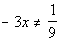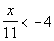TitleIntermediate Algebra
Answer/Discussion to Practice Problems
Tutorial 5: Properties of Real NumbersAnswer/Discussion to 1a  -7 The opposite of -7 is 7, since -7 + 7 = 0. The reciprocal of -7 is -1/7, since -7(-1/7) = 1. (return to problem 1a)Answer/Discussion to 1b 3/5 The opposite of 3/5 is -3/5, since 3/5 + (-3/5) = 0. The reciprocal of 3/5 is 5/3, since (3/5)(5/3) = 1. (return to problem 1b)Answer/Discussion to 2a  xy Using the commutative property of multiplication (where changing the order of a product does not change the value of it), we get  xy = yx (return to problem 2a)Answer/Discussion to 2b .1 + 3x Using the commutative property of addition (where changing the order of a sum does not change the value of it), we get .1 + 3x = 3x + .1 (return to problem 2b)Answer/Discussion to 3a (a + b) + 1.5 Using the associative property of addition (where changing the grouping of a sum does not change the value of it), we get  (a + b) + 1.5 = a + (b + 1.5) (return to problem 3a)Answer/Discussion to 3b 5(xy) Using the associative property of multiplication (where changing the grouping of a product does not change the value of it), we get  5(xy) = (5x)y (return to problem 3b)Answer/Discussion to 4a -2(x - 5)*Distribute -2 to EVERY term *MultiplyAnswer/Discussion to 4b  7(5a + 4b + 3c)*Distribute 7 to EVERY term *MultiplyAnswer/Discussion to 5a  2(x + 3) - 3x + 4*Distribute 2 through (  ) *Multiply *Reverse distributive prop with xAnswer/Discussion to 6a The difference of x and 5 is greater than or equal to 7.   Difference translates into subtraction. Is greater than or equal to will need to be replaced by the symbol >. Let's put  everything together going left to right: The difference of x and 5 is greater than or equal to 7. x - 5 > 7 (return to problem 6a)Answer/Discussion to 6b Twice the sum of y and 3 is the opposite of 10.   Twice translates as 2 times and sum converts to addition. The word is will be replaced by an =. The opposite of 10 is -10.   Let's put  everything together going left to right: Twice the sum of y and 3 is the opposite of 10. 2(y + 3) = -10 (return to problem 6b)Answer/Discussion to 6c The product of -3 and x is not equal to the reciprocal of 9.   Product translates as multiplication. Is not equal to requires the symbol. The reciprocal of 9 is 1/9.   Let's put everything together going left to right: The product of -3 and x is not equal to the reciprocal of 9.(return to problem 6c)Answer/Discussion to 6d The quotient of x and 11 is less than - 4.   Quotient translates as division. The phrase less than will be replaced by the symbol <.   Let's put everything together going left to right: The quotient of x and 11 is less than - 4.Answer/Discussion to 7a Two angles are complimentary if the sum of their measures is 90 degrees.  If the measure of one angle is x degrees, represent the measure of the other angle as an expression of x.  Let's think about this for a second. Let's pretend that one of our angles is 70 degrees.  What would be the measure of the other angle if they had to add to be 90?  If you said 20, you are correct!  The thought behind how we got that 20 is, the total of 90 is given, we have already used 70, so we would subtract 70 from 90 and that would tell us what the complimentary angle would have to be.  So, using x, we can write an expression that shows that we take the total of 90 and subtract the given angle of x: 90 - x (return to problem 7a)Answer/Discussion to 8a  ½   ?  .5 Since ½ written in decimal form is .5, then 1/2 equals .5: ½ = .5 (return to problem 8a)Answer/Discussion to 8b  -3.5   ?   1.5 Since -3.5 is to the left of 1.5 on the number line, then -3.5 is less than 1.5: -3.5 < 1.5 (return to problem 8b)Answer/Discussion to 8c 4.3    ?   4.21 Since 4.3 is to the right  of  4.21 on the number line, then 4.3 is greater than 4.21: 4.3 >  4.21 (return to problem 8c)

Last revised on June 11, 2011 by Kim Seward.
All contents copyright (C) 2002 - 2011, WTAMU and Kim Seward. All rights reserved.